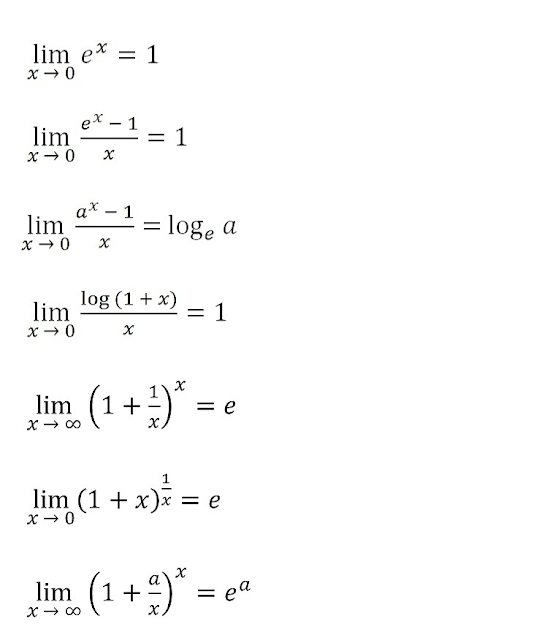# Limits and Continuity: Class 11 Mathematics Solutions | Exercise - 15.2## Chapter - 15  Limits and Continuity

In this chapter, we are basically going to learn about the methods of finding Limits trigonometric functions. We have already learned how can we find limits of normal function in previous exercise. If you haven't completed the previous exercise then you should have a look at that exercise before moving forward.

As, I have already informed you that in this exercise we are going to find the limits of trigonometric functions. So inorder to find the limit of trigonometric functions, you need to know some basic trigonometry formula.

### Trigonometry Formula

We need to remember trigonometry formula that we have read in class 10. We need them while solving the problems given in this exercise.  If you do remember all the formula then that's great for you. Even if you don't remember them,  don't you worry.  I have listed all the formula needed to finish this exercise. So have a look at some of the necessary trigonometry formulas.

• Sin(A+B) = sinA.cosB + cosA.sinB
• Sin (A-B) = sinA.cosB - cosA.sinB
• Cos(A + B) = cosA.cosB - sinA.sinB
• Cos(A - B) = cosA.cosB + sinA.sinB
• sin 2A = 2sinA.cosA
• Cos2A = 2cos²A - 1 or 1- 2sin²A
• 1 - cos 2A = 2sin²A
• 1 - cosA = 2sin²A/2
• 1 + cos2A = 2cos²A
• 1 + cosA = 2Cos²A/2
• sinC + sinD = 2sin (C+D)/2×cos(C-D)/2
• sinC - sin D = 2cos (C+D)/2×sin(C-D)/2
• cosC+ cosD = 2cos (C + D)/2×cos (C-D)/2
• cosC- cosD= 2sin(C+D)/2×sin(D-C)/2
• cos²A - sin²A =cos2A

Well these are some of the formula that we already have learned in grade 10 previous year.

### Limits Of Trigonometric Functions

Apart from previous year trigonometry formula, we need to remember some extra formula this year in grade 11. This formula are known as limit formula for trigonometric functions.  Some of the important formula are given below;

### Limits Log and Exponential Functions

We also need formula of log and exponential function while finding their limits. Some of the necessary formula to find the limits of log and exponential functions are given below;### Exercise - 15.2

This PDF contains all the solutions of class 11, Limits and Continuity Chapter. It only contains solutions of exercise - 15.2. If you want solutions of exercise - 15.1 and exercise -  15.3 then you'll find them above. You just need to click on the button given above.

NoteScroll the PDF to view all Solution

You are not allowed to post this PDF in any website or social platform without permission.

## Is Class 11 Mathematics Guide Helpful For Student ?

I created these notes to assist students who struggle to solve challenging math problems. The student should not completely rely on this note to finish all of the exercises. It may affect your study if you rely solely on this note and simply copy it as written.

Students should also try to solve problems themselves by using their own willpower. You can utilize this math guide PDF as a kind of perspective. Before copying, you should make sure that all of the answers are correct. The note may contain some minor errors; please take those errors into consideration.

## How to secure good marks in Mathematics ?

You may be aware that I am also a student. It's hard to be a student. You have to study multiple subjects at the same time. The majority of students, in my opinion, lack mathematical proficiency. You can use my weakness in mathematics as an illustration. When I attempt to solve math problems, I also encounter difficulties.

You should practice them every day if you want to get good grades in mathematics. You should go over every exercise that was taught in class once more. Start with simple questions that you already know to solve math problems. You won't get bored if you do this.

Math is more than just practicing; especially in 11th grade, you need to understand the problem's fundamentals. When you understand the problem's fundamental idea, you can quickly identify other problems with a similar approach.

Every student tries to remember the same example when your teacher tries to make the concept clear by giving examples, but you should never do that. You can come up with your own formula that you won't forget in the future.

You can definitely get good grades on your exam if you practice properly and give yourself enough time to do so.

Disclaimer: This website is made for educational purposes. If you find any content that belongs to you then contact us through the contact form. We will remove that content from our as soon as possible.

If you have any queries then feel free to comment down.
No Comment